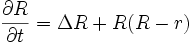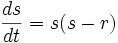# Scalar curvature equation

## Definition

The scalar curvature equation is a second-order differential equation that describes the evolution of the scalar curvature associated with the Riemannian metric on a differential manifold, under the volume-normalized Ricci flow.

The scalar curvature equation is as follows:$\frac{\partial R}{\partial t} = \Delta R + R(R-r)$

Here$r$ denotes the average scalar curvature. In the particular case of a compact orientable surface,$r$ is a constant computable using the Gauss-Bonnet theorem, and is independent of$t$.

## Analysis of the scalar curvature term

### As a reaction-diffusion equation

The scalar curvature equation is a classical example of a reaction-diffusion equation, that is, it describes a flow where the reaction term is trying to concentrate the curvature at some points, while the diffusion term is trying to equalize, or diffuse, the curvature. Thus, we can apply a suitable maximum principle here and study bounds on the PDE in terms of the associated ordinary differential equation for the reaction term:$\frac{ds}{dt} = s(s-r)$

We can solve this in an exact manner and obtain:$s(t) = \frac{r}{1 - (1 - r/s_0)e^{rt}}$

This immediately gives us some lower bounds for the scalar curvature at all times.